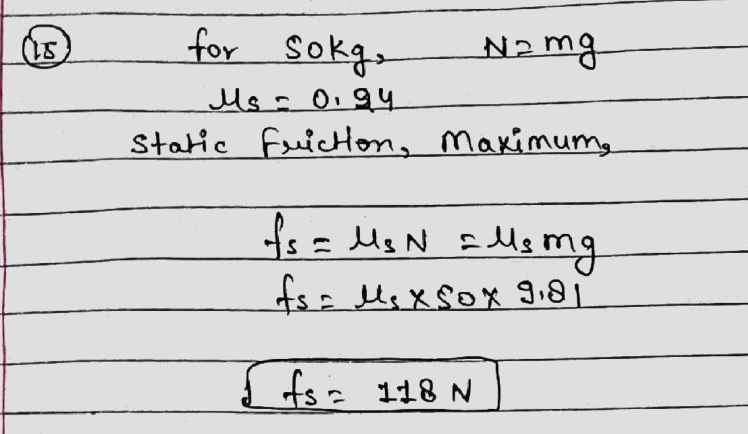Question

Statics

What is the maximum value of the static friction? (N)Verified### Question 45473Statics

6. Particles A and B start from rest at the origin, s = 0, and move along a straight line with acceleration a+6t-3 fts2 and a(12t2-8)srespectively. Determine the distance between the two particles at t = 4s and the total distance each particle has travelled at t=4s

### Question 45472Statics

5. Little Johnny throws a baseball straight upwards with a speed of 15 m/s.Once it has left his hand, the acceleration of the ball is 9.81 m/s towards the Earth. Determine the amount of time it takes for the ball to return to its original position.

### Question 45471Statics

4. Travelling with a speed of 35 mile/hr, a car begins to accelerate at 3000mile/hr along a straight road. How long will it take to reach a speed of 60mile/hr? How much distance does the car travel during this time?

### Question 45470Statics

3. A particle travels along a straight line with an acceleration of a = (9t – 10)m/s?, where t is in seconds. What is the particle's velocity when t =4s, and what is its position when t = 10 s if the particle starts from rest?

### Question 45469Statics

2. A particle travels along a straight line with an acceleration of a (20-0.4)=m/s?, where s is measured in meters. Determine the velocity of the particle when s = 8 m if v = 3 m/s at s = 0.

### Question 45468Statics

1. The position of a particle which moves along a straight line is described by the equation s =t3-9t2+15tequations which describe the velocity and the acceleration of the particle.State the particle's velocity and acceleration at t=8

### Question 45236Statics

Find the forces in the members of the bridge truss shown below.

### Question 45235Statics

) Determine the magnitude and direction q of F so that the practical is in equilibriur

### Question 45234Statics

For the concurrent force system shown in the figure, find the resultant force-it's magnitude and it's rectangular components.

### Question 45041Statics

For a short time the arm of the robot is extending1.5 ft/s when r=such that r3 ft, z =(47) ft, and= A0.5t rad, where t is in seconds. Determine the magnitudes of the velocity and acceleration of the grip A when t = 3 s.

### Submit query

Getting answers to your urgent problems is simple. Submit your query in the given box and get answers Instantly.

### Submit a new QuerySuccess

Assignment is successfully created